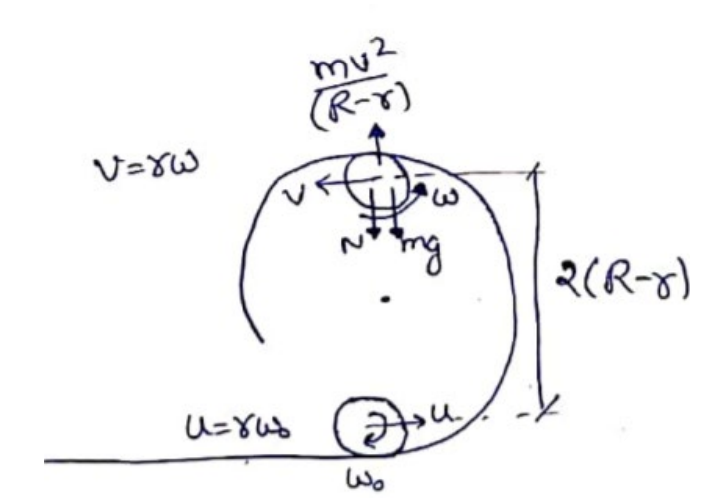Deepak Scored 45->99%ile with Bounce Back Crack Course. You can do it too!

# Solve the following :

Question:

Figure shows a rough track, a portion of which is in the form of a cylinder of radius $\mathrm{R}$. With what minimum linear speed should a sphere of radius $r$ be set rolling on the horizontal part so that it completely goes round the circle on the cylindrical part.

Solution:For minimum u, the ball will just be in contact with surface at top. So, $\mathrm{N}=0$

$m g=\frac{m v^{2}}{(R-r)}$

$v^{2}=g(R-r)$

Now, by energy conservation $\frac{1}{2} m u^{2}+\frac{1}{2} I \omega_{0}^{2}=\frac{1}{2} m v^{2}+\frac{1}{2} I \omega^{2}+m g \cdot 2(R-r)$

$\frac{1}{2} m u^{2}+\frac{1}{2}\left(\frac{2}{5} m r^{2}\right)\left(\frac{u^{2}}{r^{2}}\right)=\frac{1}{2} m g(R-r)+\frac{1}{2}\left(\frac{2}{5} m r^{2}\right)\left(\frac{g(R-r)}{r^{2}}\right)+2 m g(R-r)$

$u=\sqrt{\frac{27}{7} g(R-r)}$